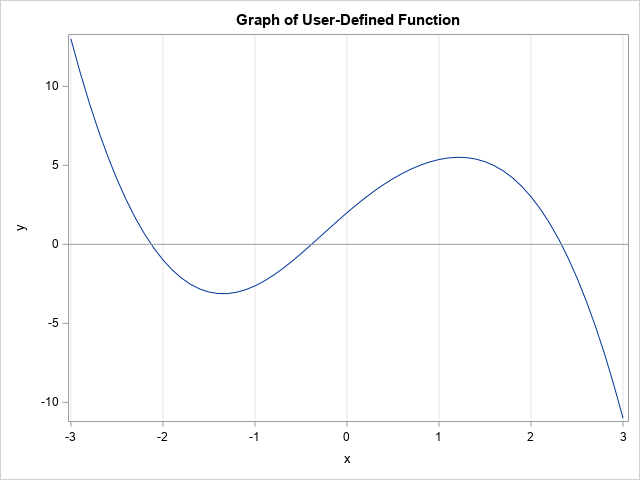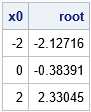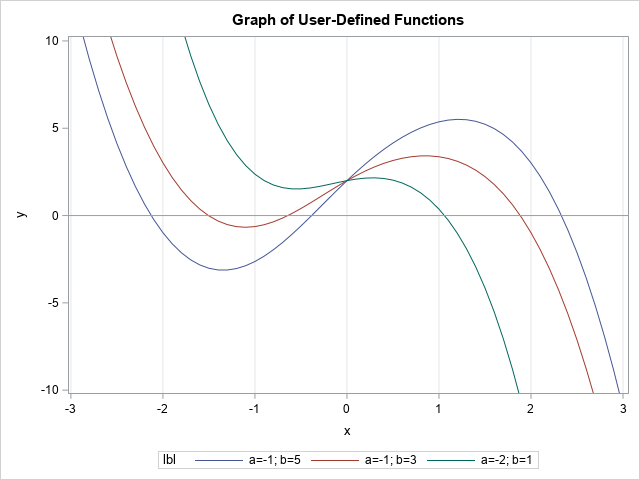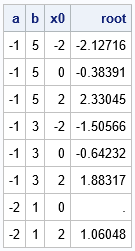Finding the root (or zero) of a nonlinear function is an important computational task. In the case of a one-variable function, you can use the SOLVE function in PROC FCMP to find roots of nonlinear functions in the DATA step. This article shows how to use the SOLVE function to find the roots of a user-defined function from the SAS DATA step.

### Some ways to find the root of a nonlinear function in SAS

I have previously blogged about several ways to find the roots of nonlinear equations in SAS, including:

The DATA step does not have a built-in root-finding function, but you can implement one by using PROC FCMP.

### PROC FCMP: Implement user-defined functions in SAS

PROC FCMP enables you to write user-defined functions that can be called from the DATA step and from SAS procedures that support DATA step programming statements (such as PROC NLIN, PROC NLMIXED, and PROC MCMC). To demonstrate finding the roots of a custom function, let's use the same function that I used to show how to find roots by using SAS/IML. The following statements use PROC FCMP to define a function (FUNC1) and to store the function to WORK.FUNCS. You must use the CMPLIB= system option to tell the DATA step to look in WORK.FUNCS for unknown functions. The DATA step evaluates the function for input arguments in the interval [-3, 3]. The SGPLOT procedure creates a graph of the function:

```/* use PROC FCMP to create a user-defined function (FUNC1) */ proc fcmp outlib=work.funcs.Roots; function Func1(x); /* create and store a user-defined function */ return( exp(-x**2) - x**3 + 5*x +1 ); endsub; quit; options cmplib=work.funcs; /* DATA step will look here for unresolved functions */   data PlotIt; do x = -3 to 3 by 0.1; /* evaluate function on [-3, 3] */ y = Func1(x); output; end; run;   title "Graph of User-Defined Function"; proc sgplot data=PlotIt; series x=x y=y; refline 0 / axis=y; xaxis grid; run;```From the graph, it appears that the function has three roots (zeros). The approximate locations of the roots are {-2.13, -0.38, 2.33}, as found in my previous article. The next section shows how to use the SOLVE function in PROC FCMP to find these roots.

### Use the SOLVE function to find roots

The SOLVE function is not a DATA step function. It is a "special function" in PROC FCMP. According to the documentation for the special functions, "you cannot call these functions directly from the DATA step. To use these functions in a DATA step, you must wrap the special function inside another user-defined FCMP function." You can, however, call the SOLVE function directly from procedures such as NLIN, NLMIXED, and MCMC.

I was confused when I first read the documentation for the SOLVE function, so let me give an overview of the syntax. The SOLVE function enables you to find a value x such that f(x) = y0 for a "target value", y0. Thus, the SOLVE function enables you to find roots of the function g(x) = f(x) – y0. However, in this article, I will set y0 = 0 so that x will be a root of f.

Because the function might have multiple roots, you need to provide a guess (x0) that is close to the root. The SOLVE function will start with your initial guess and apply an iterative algorithm to obtain the root. Thus, you need to provide the following information to the SOLVE function:

• The name of an FCMP function (such as "Func1") that will evaluate the function.
• An initial guess, x0, that is near a root. (You can also provide convergence criteria that tells the iterative algorithm when it has found an approximate root.)
• The parameters to the function. A missing value indicates the (one) parameter that you are solving for. For a function of one variable, you will specify a missing value for x, which tells the SOLVE function to solve for x. In general, a function can take multiple parameters, so use a missing value in the parameter list to indicate which parameter you are solving for.

The following SAS program defines a new FCMP function called Root_Func1. That function takes one argument, which is the initial guess for a root. Inside the function, the SOLVE function finds the root of the "Func1" function (defined earlier). If it was successful, it returns the root to the caller. To test the Root_Func1 function, I call it from a DATA step and pass in the values -2, 0, and +2. I obtained these guesses by looking at the graph of the Func1 function.

```/* to call SOLVE in the DATA step, wrap it inside an FCMP function */ proc fcmp outlib=work.funcs.Roots; function Root_Func1(x0); array opts init absconv relconv maxiter status; /* initialize to missing */ opts = x0; /* opts is the initial guess */ z = solve("Func1", /* name of function */ opts, /* initial condition, convergence criteria, and status */ 0, /* target: find x so that f(x) = 0 */ .); /* Params for f. A missing value indicates param to solve for */ if status=0 then return( z ); /* root found */ else return (.); /* a root was not found */ endsub; quit;   data Roots1; input x0 @@; root = Root_Func1(x0); /* x0 is a "guess" for a root of Func1 */ datalines; -2 0 2 ;   proc print data=Roots1 noobs; run;```The output shows that the function has found three roots, which are approximately {-2.13, -0.38, 2.33}. These are the same values that I found by using the FROOT function in SAS/IML.

### The roots of functions that have parameters

Sometimes it is useful to include parameters in a function. For example, the following function of x contains two parameters, a and b:
g(x; a, b) = exp(-x2) + a*x3 + b*x +1
Notice that the first function (Func1) is a special case of this function because f(x) = g(x; -1, 5). You can include parameters in the FCMP functions that define the function and that find the roots. When you call the SOLVE function, the last arguments are a list of parameters (x, a, b) and you should give specific values to the a and b parameters and use a missing value to indicate that the x variable is the variable that you want to solve for. This is shown in the following program:

```/* you can also define a function that depends on parameters */ proc fcmp outlib=work.funcs.Roots; /* define the function. Note Func1(x) = Func2(x; a=-1, b=5) */ function Func2(x, a, b); return( exp(-x**2) + a*x**3 + b*x +1 ); endsub;   function Root_Func2(x0, a, b); array opts init absconv relconv maxiter status; /* initialize missing */ init = x0; /* pass in initial guess */ z = solve("Func2", /* name of function */ opts, /* initial condition, convergence opts, status */ 0, /* Find x so that f(x) = 0 */ ., a, b ); /* Params for f. A missing value indicates param to solve for */ if status=0 then return( z ); /* root found */ else return (.); /* a root was not found */ endsub; quit;   /* Evaluate Func2 for x in [-3,3] for three pairs of (a,b) values: (-1,5), (-1,3), and (-2,1) */ data PlotIt; do x = -3 to 3 by 0.1; y = Func2(x, -1, 5); lbl="a=-1; b=5"; output; y = Func2(x, -1, 3); lbl="a=-1; b=3"; output; y = Func2(x, -2, 1); lbl="a=-2; b=1"; output; end; run;   title "Graph of User-Defined Functions"; proc sgplot data=PlotIt; series x=x y=y / group=lbl; refline 0 / axis=y; xaxis grid; yaxis min=-10 max=10; run;```The three graphs are shown. You can see that two of the graphs have three roots, but the third graph has only one root. You can call the Root_Func2 function from the DATA step to find the roots for each function:

```/* find the roots of Func2 for (a,b) values (-1,5), (-1,3), and (-2,1) */ data Roots2; input a b x0; root = Root_Func2(x0, a, b); datalines; -1 5 -2 -1 5 0 -1 5 2 -1 3 -2 -1 3 0 -1 3 2 -2 1 0 -2 1 2 ;   proc print data=Roots2 noobs; run;```Notice that the roots for (a,b) = (-1, 5) are exactly the same as the roots for Func1. The roots for the function when (a,b) = (-1, 3) are approximately {-1.51, -0.64, 1.88}. The root for the function when (a,b) = (-2, 1) is approximately 1.06.

Notice that the initial guess x0 = 0 did not converge to a root for the function that has (a,b) = (-2, 1). It is well known that Newton-type methods do not always converge. This is in contrast to Brent's methods (used by the FROOT function in SAS/IML), which always converges to a root, if one exists.

### Summary

In summary, this article shows how to use the SOLVE function in Base SAS to find the roots (zeros) of a nonlinear function of one variable. You first define a nonlinear function by using PROC FCMP. You can call the SOLVE function directly from some SAS procedures. However, if you want to use it in the DATA step, you need to define a second FCMP function that takes the function parameters and an initial guess and calls the SOLVE function. This article shows two examples of using the SOLVE function to find roots in SAS.

The post Find the root of a function by using the SAS DATA step appeared first on The DO Loop.